Get instant live expert help with Excel or Google Sheets“My Excelchat expert helped me in less than 20 minutes, saving me what would have been 5 hours of work!”

#### Post your problem and you’ll get Expert help in seconds.

Your message must be at least 40 characters
Our professional Expert are available now. Your privacy is guaranteed.

# How to Make VLOOKUP Reference Left Column in Excel

While using the standard VLOOKUP function in Excel, a lookup value must be in the leftmost column of a table. In this tutorial, we will learn how to look up for the value even if it is not in the leftmost. In order to do this, we can use the combination of INDEX and MATCH functions or VLOOKUP and CHOOSE function.

## Syntax of INDEX and MATCH functions

Firstly, we will explain how to use both of these functions separately. INDEX function returns a value of a particular row in an array. The generic formula looks like:

`=INDEX(array, row_num, [col_num])`

The parameters of the INDEX function are:

• array – an array in which we want to find a value in a certain position
• row_num – a row number in an array from which we want to take a value
• col_num – a column number in an array from which we want to take a value. This is a non-mandatory parameter.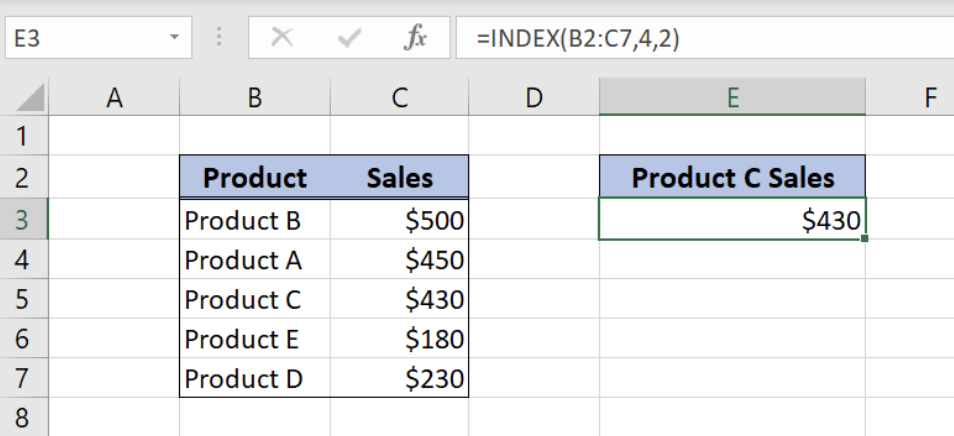Figure 1. Get the Product C Sales Data from the Table with INDEX function

The MATCH function returns a position of a certain value in an array. The generic formula looks like:

`=MATCH(lookup_value, lookup_array, [match_type])`

The parameters of the MATCH function are:

• lookup_value – a value which position in an array we want to get
• lookup_array – an array in which we want to find a position of a value
• match_type – a type of match which will be returned. We will use 0 because we want the position of an exact matching value.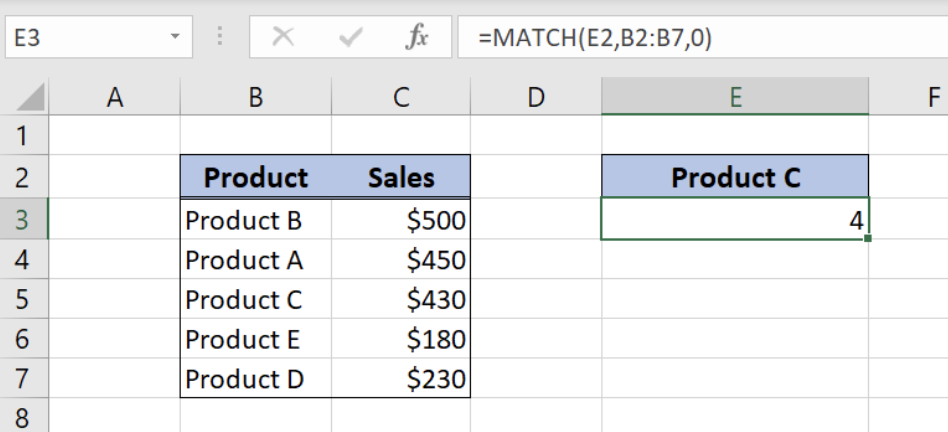Figure 2. Get the Row Number from the Table Column with MATCH Function

Now, we can look at the generic formula which allows us to look up reference in the left column:

`=INDEX(array, MATCH(lookup_value, lookup_array, 0))`

The idea is to find a position of a value which we want to find in the lookup array by the MATCH function. Then, we will find a value of that position in the value’s array by INDEX function.

We will now look at the example to explain in details how this formula works. Let’s start with examining the structure of the data that we will use.

The lookup table consists of 3 columns:

• The first column is “Sales” (B),
• The second one is “Product” (C),
• While the third one is “Salesman” (D).

The main table where we want to get data consists of 2 columns:

• The first column is “Product (F),
• The second one is “Sales” (G).

As a result, we want to get Sales value from column B into “Sales” column G, based on “Product” columns match.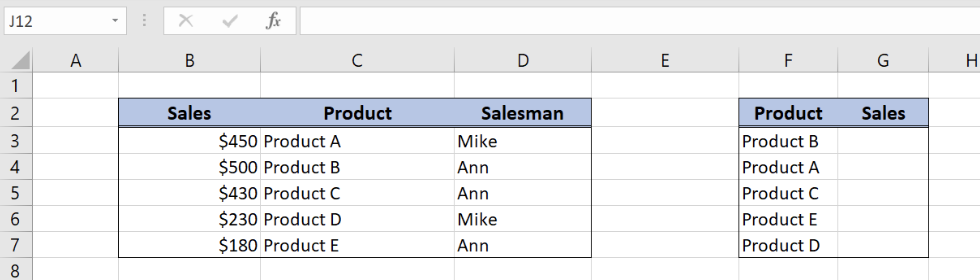Figure 3. Tables for INDEX and MATCH lookup

## Get the Product Sales with INDEX and MATCH

The formula for getting data from the table where lookup array is on the right side looks like:

`=INDEX(\$B\$3:\$B\$7,MATCH(F3,\$C\$3:\$C\$7,0))`

Let’s look into the MATCH function parameters:

• lookup_value – “Product B” (F3 cell)
• lookup_array – “Product” column in the first table (C3:C7)
• match_type – 0, exact match

As a result of the MATCH function, we will get value 2, as “Product B” is the second in array C3:C7. This value is row_num parameter for the INDEX function.

INDEX function parameters in the example are:

• array – column “Sales” from the first table (B3:B7)
• row_num – result from the match function (value 2)

Column “Sales” from the first table is array in INDEX because we want to get the value of sales from position 2.

To use INDEX and MATCH functions to reference left column, we need to follow these steps:

• Select cell G3 and click on it
• Insert the formula: `=INDEX(\$B\$3:\$B\$7,MATCH(F3,\$C\$3:\$C\$7,0))`
• Press enter
• Drag the formula down to the other cells in the column by clicking and dragging the little “+” icon at the bottom-right of the cell.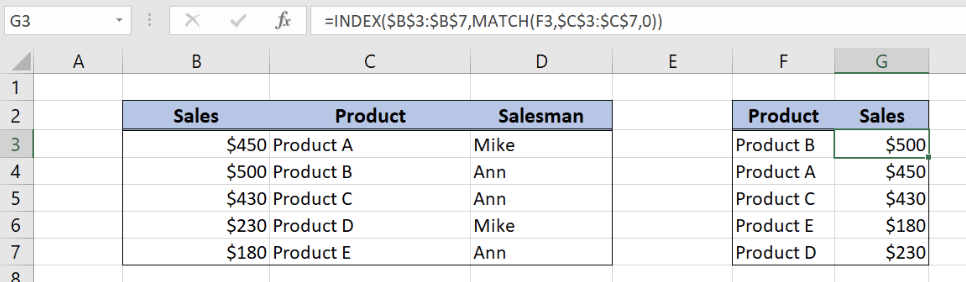Figure 4. INDEX and MATCH lookup

As a result, we will get \$500 in the G3 cell, as “Product B” has position 2 in array C3:C7. Furthermore, position 2 in array B3:B7 has value \$500, which is the result of our formula.

## An application of VLOOKUP and CHOOSE functions

Firstly, we will explain how to use both of these functions separately. VLOOKUP function looks up for the value and returns the matching value.

The generic formula for VLOOKUP looks like:

`=VLOOKUP(lookup_value, table_array, col_index_num, range_lookup)`

The parameters of the VLOOKUP function are:

• lookup_value – a value which we want to find in a lookup table
• table_array: – a table in which we want to look up.
• col_index_num – a column number in a lookup table which value we want to pull
• range_lookup – default value 0. This means that we want to find an exact match for a lookup value.

The CHOOSE function can form a table based on given values and positions. First we will explain how the single CHOOSE function works, and how can we use it to change column places in the table.

The generic formula for CHOOSE looks like:

`=CHOOSE(index_num, value1, value2, ..., valueN)`

The parameters of the CHOOSE function are:

• index_num – position of the value that we want to get
• value1, value2, … – The first, second etc. value from which to choose.

Excel CHOOSE function returns the value from the list, we just have to define a position of that value in the value list.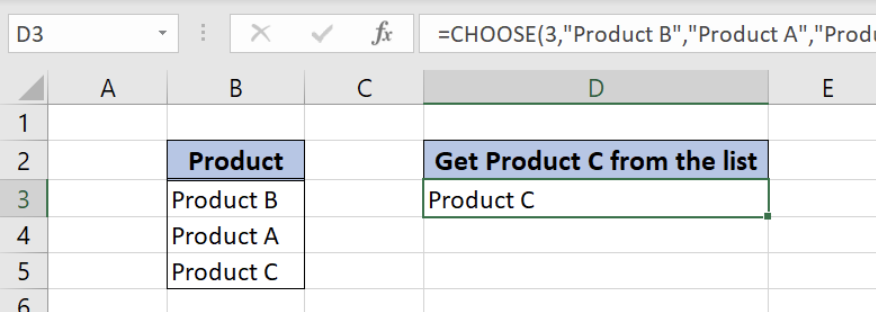Figure 5. Get the Product C from the table with CHOOSE

For our problem, single CHOOSE function is not very usable. We can use CHOOSE function in combination with VLOOKUP to get the value from the table where lookup array column is on the right side.

## Get the value from the table with VLOOKUP and CHOOSE

The idea is to create a table by switching columns position, using CHOOSE function. As a result, the new table (with swapped columns) will be the table_array parameter for VLOOKUP function).

The generic formula which allows us to lookup reference in left column is:

`=VLOOKUP(lookup_value, CHOOSE({1,2}, value1, value2), col_index_num, 0)`

We will now look at the example to explain in details how this function works. The data structure is the same as in Example 1.

As a result, we want to get Sales value from column B into “Sales” column G, based on “Product” columns match. The idea is to swap positions of columns “Product” and “Sales” in the first table by CHOOSE function. After that, we can use VLOOKUP function in a standard way.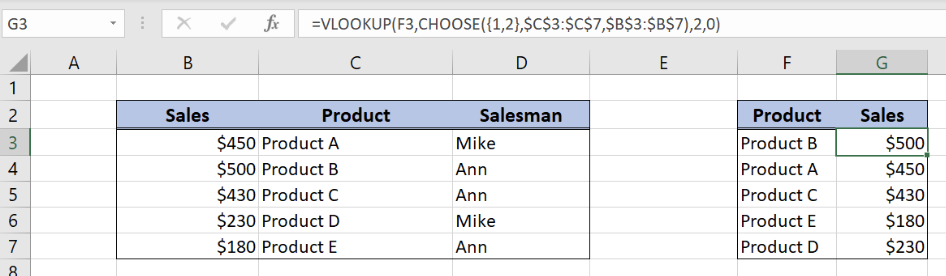Figure 6. VLOOKUP and CHOOSE lookup

The formula looks like:

`=VLOOKUP(F3, CHOOSE({1,2}, \$C\$3:\$C\$7, \$B\$3:\$B\$7), 2, 0)`

• In order to use columns B and C in VLOOKUP, we will swap their positions by CHOOSE function
• The parameter index_num of CHOOSE function has value {1,2}, while the C3:C7 range is value1 and B3:B7 is value2.
• Don’t forget to put index_num values in brackets {} because we are dealing with arrays

This means that the column “Product” will become the first column in the table and the column “Sales” will become the second. A table like this will be the table_array parameter of the VLOOKUP function.

• In VLOOKUP formula, lookup_value is “Product B” (F3 cell).
• Lookup_array is the table from CHOOSE function
• Col_index_num has value 2, as we want to pull value from the second column of the range.
• Finally, range_lookup has value 0, because we want to find an exact match of “Product” values.

To use VLOOKUP and CHOOSE functions to reference left column, we need to follow these steps:

• Select cell G3 and click on it
• Insert the formula: `=VLOOKUP(F3, CHOOSE({1,2}, \$C\$3:\$C\$7, \$B\$3:\$B\$7), 2, 0)`
• Press enter
• Drag the formula down to the other cells in the column by clicking and dragging the little “+” icon at the bottom-right of the cell.

As a result, we will get \$500 in the G3 cell, as “Product B” has position 2 in array C3:C7. Furthermore, position 2 in array B3:B7 has value \$500, which is the result of our formula.

Most of the time, the problem you will need to solve will be more complex than a simple application of a formula or function. If you want to save hours of research and frustration, try our live Excelchat service! Our Excel Experts are available 24/7 to answer any Excel question you may have. We guarantee a connection within 30 seconds and a customized solution within 20 minutes.

Are you still looking for help with the VLOOKUP function? View our comprehensive round-up of VLOOKUP function tutorials here.

### Did this post not answer your question? Get a solution from connecting with the expert.Another blog reader asked this question today on Excelchat:
Solution examplesindex and match with duplicates. I need to use another column as a reference, so my return value has two match the value of two things for it to return
Solved by E. H. in 60 minsI have a file with two sheets. On the 1st, I have dollars in O13and in O16 a number signifying 1-6 people. On the second workbook is a matrix that corresponds to the dollar amount AND the number of people. For example, sheet 1 O13 is \$6,000. O16 is 3. I want a formula to find \$6,000 (not higher) on sheet 2 in column A. Then I want it to compare sheet 1 O16 to the correct column (B-G representing 1-6) on sheet 2 for an exact match to place in sheet1 O17. The range of sheet1 O13 is referenced on sheet2 A5-A383, and sheet 1 O16 is referenced on sheet2 B5:G383. I have an INDEX Match but it isn't quite working, yet.
Solved by B. B. in 60 minsI have two lists. One is a list of part numbers. The other is a list of long text descriptions. I can use VLOOKUP with "*"&cell address"*" to find the first match. But how can I find the second and third matches? I have tried using INDEX and SHORT but the wild cards no longer find the match like they do in VLOOKUP. Can you help?
Solved by S. J. in 36 minsHello, I have a big spreadsheet in which I need to know how many patients came in each month based on provider. I am using the following formula but I still get the N/A error. =INDEX('No Show Appts Data'!D:E, MATCH(1, 'No Show Appts Data'!D:D='No Shows Data'!M2)*('No Shows Data'!E:E='No Shows Data'!N1),0)) No Show Appts Data is the name of the sheet where Column D is Month Year of date in question and Column E is the provider. No Shows Data is the sheet where I am making all the formula calculation where Column M is Month and Year and Column N is the provider in question and therefore M2 is the month in question and N1 the provider in question. How do I fix this error? Thanks
Solved by F. H. in 40 minsI need to find an INDEX function that will convert the Call Day (a number 1-7) to the actual weekday found in row 1 of the DayofWeek named range. I don't know if I'm supposed to use the MATCH function as well or not.
Solved by D. D. in 11 mins## Subscribe to Excelchat.coAnother blog reader asked this question today on Excelchat: